## Меню

Главная
Финансы деньги и налоги
Издательское дело и полиграфия
Новейшая история политология
Топики по английскому языку
Языкознание филология
Химия
Экология и охрана природы
Естествознание
Искусство и культура
Культурология
Хозяйственное право
Иностранные языки и языкознание
История и исторические личности
Коммуникации связь цифровые приборы и радиоэлектроника
Товароведение
Транспорт
Экология охрана труда
Банковское и биржевое дело
Коммуникации и связь
Конституционное право
Этика

##### Научно-образовательный портал W-10.RU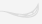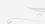Главная

# Mathematical Formulas for Calculation of Newtonian Constant of Gravitation G

How Many Physical Constants are Truly Fundamental? Dr. Nikolay Kosinov Annotation

To main fundamental constants scientists usually refer gravitational constant (G), Planck constant (h) and speed of light (c). These constant are considered to be independent. Researches have shown, that truly fundamental are not constants G, h, c, but quite other constants [1, 2, 3, 4]. They are the following constants:

1. Fundamental quantum of action hu (hu=7,69558071(63) • 10-37 J s).

2. Fundamental length lu (lu=2,817940285(31) • 10-15 m).

3. Fundamental quantum of time tu (tu=0,939963701(11) • 10-23 s).

4. Fine structure constant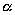(=7,297352533(27) • 10-3 )

5. Number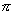(=3,141592653589).

All major fundamental physical constants are composed and consist of these five constant. These five constant have special status and are called "universal superconstants" [1, 2, 3, 4].

Are the major physical constants fundamental?

To main fundamental constant scientists usually refer gravitational constant (G), Planck constant (h) and speed of light (c). These constant are considered to be independent. These physical constants have special status among other constant. It is confirmed by the fact, that in fundamental physics many scientists used the units system, in which these constants equal 1. They received a special importance after M.Planck-s researches, who with the help of their combination discovered the units of mass, length and time, which were called "Planck units".

Constants G, h, c and their different combinations are used in most important physical theories. For example, Newtonian theory of gravitation is G-theory . General theory of relativity is a classic (G, c)-theory. Relative quantum theory of field is quantum (h, c)-by theory . Each of these theories operates by one or two dimensional constants. The discovery of Planck units v Planck length, mass and time - gave a hope, that it is possible to create a new quantum theory on the base of three constants G, h, c. But attempts to create a single quantum theory of electromagnetic fields, particles and gravitation on the base of three dimensional constants G, h, c were unsuccessful. This theory has not existed yet, although scientists showed promises of it . Why did it happen? The reason is, that constants G, h, c can not be a constant base of quantum theory. In accordance with this fact a question arises, whether these constants can be considered primary and independent? Difficulties in creation of G, h, c -theories object it. Obviously, other constant exist, which are both independent, and primary, and truly fundamental. Evidently, all basic physical constants should come of such primary constants, including constants G, h, c. Since primary status of constants G, h, c was out doubts for a long time, the task of search of ontological base of fundamental physical constant does not arise so sharp.

Failures in creation of (G, h, c)-theories and a big number of other fundamental physical constants, among which it is difficult to prefer one of them, bring forward s task of search of ontological base of physical constants. Contemporary physics accumulated already about 300 fundamental constants . 300 constants and all are fundamental! Why such a big number of constants are considered to be fundamental? If we refer them to truly fundamental constants, it exists too much of them. If we accept a fact, that a single material essence makes a world base and all of physical phenomena should have a single nature, then amount of constants must be lesser. Here we remember Ockham-s rule, according to which we should not multiply a number of essences without necessity, and also Fresnel opinion, that L the nature is disposed to operate by great things with the help of the little¦ [5, 8]. Therefore, if priority and independence of constants are criterions of true fundamentality, then just a little amount of constants should be considered to be truly fundamental. That is why, a deep contradiction exists in fact, that hundreds of constants have fundamental status. Our task is to find among them "truly fundamental constants" and reveal a number of them. Many facts indicate, that it is sufficient to have three dimensional constants as truly fundamental. However only three basic units - metre, kilogramme and second v are enough to receive all units, having mechanical nature. But unsuccessful attempts in creation of (G, h, c)-theories show, that three constants are not enough. It means, that we should search the unknown number JF, which comply with a number of still unknown truly fundamental constant, should be searched somewhere between 3 and 300:

3 < JF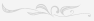## Поиск

Расширенный поиск